# Algebra II : Using Probability to Make Decisions

## Example Questions

← Previous 1

### Example Question #1 : Using Probability To Make Decisions

There are two raffles that both support causes that you care about. You have $20, and want to purchase a ticket for the raffle that gives you the best odds of winning. Raffle A supports a local charity, and has 75 tickets. Each ticket costs$15. One ticket will win a $110 prize, and the remaining tickets will win nothing. Raffle B supports a national charity, and has 100 tickets. Each ticket costs$20. One ticket will win a $410 prize, and the remaining tickets will win nothing. Which raffle is a better deal? Possible Answers: Raffle A, with payoff of -$13.57

Raffle A, with payoff of -$15.90 Raffle B, with payoff of -$13.57

Raffle B, with payoff of -$15.90 Correct answer: Raffle A, with payoff of -$13.57

Explanation:
To solve this problem, first calculate the expected profit of raffle A. The expected profit is the expected payoff minus the cost. Since it costs $15 to enter the raffle, the cost is$15. Next, let X be a random variable whose value is the payoff in the raffle. Since the only prize is $110, the values X can take are$110 and $0 (if no prize is won). Since 1 ticket has a payoff of$110, the probability of winning $110 is 1/75 = 0.013. In other words P($110)=.013. Since the 74 remaining tickets have a payoff of $0, the probability of winning$0 is P($0)=.987. To find E(X), the expected value of X, find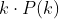for each value k that X can take. Then sum these terms.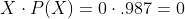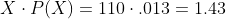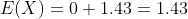Therefore, the expected payoff is$1.43. The expected profit of Raffle A is the expected payoff minus the cost: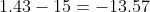Next, let's look at Raffle B. The cost of Raffle B is $20. Then, we want to find the expected payoff for Raffle B. This time, we'll use the random variable Y. Since the only prize is$410, the values X can take are $410 and$0 (if no prize is won). Since 1 ticket has a payoff of $410, the probability of winning$410 is 1/100 = 0.01. In other words P($410)=.01. Since the 99 remaining tickets have a payoff of$0, the probability of winning $0 is P($0)=.99.

To find E(Y), the expected value of Y, findfor each value k that Y can take. Then sum these terms.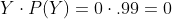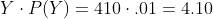Therefore, the expected payoff is $4.10. The expected profit of Raffle B is the expected payoff minus the cost: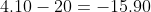Since -$13.57 > -$15.90, Raffle A has the better payoff. ### Example Question #71 : Probability There are two raffles that both support causes that you care about. You have$20, and want to purchase a ticket for the raffle that gives you the best odds of winning.

Raffle A supports a local charity, and has 100 tickets. Each ticket costs $17. One ticket will win a$250 prize, and the remaining tickets will win nothing.
Raffle B supports a national charity, and has 200 tickets. Each ticket costs $19. One ticket will win a$290 prize, and the remaining tickets will win nothing.

Which raffle is a better deal?
Possible Answers:

Raffle B, with payoff of -$14.50 Raffle A, with payoff of -$17.55

Raffle A, with payoff of -$14.50 Raffle B, with payoff of -$17.55

Correct answer:

Raffle A, with payoff of -$14.50 Explanation: To solve this problem, first calculate the expected profit of raffle A. The expected profit is the expected payoff minus the cost. Since it costs$17 to enter the raffle, the cost is $17. Next, let X be a random variable whose value is the payoff in the raffle. Since the only prize is$250, the values X can take are $250 and$0 (if no prize is won). Since 1 ticket has a payoff of $250, the probability of winning$250 is 1/100 = 0.01. In other words P($250)=.01. Since the 99 remaining tickets have a payoff of$0, the probability of winning $0 is P($0)=.99.

To find E(X), the expected value of X, findfor each value k that X can take. Then sum these terms.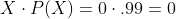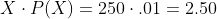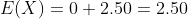Therefore, the expected payoff is $2.50. The expected profit of Raffle A is the expected payoff minus the cost: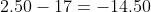Next, let's look at Raffle B. The cost of Raffle B is$19. Then, we want to find the expected payoff for Raffle B. This time, we'll use the random variable Y. Since the only prize is $290, the values X can take are$290 and $0 (if no prize is won). Since 1 ticket has a payoff of$290, the probability of winning $290 is 1/200 = 0.005. In other words P($290)=.005. Since the 199 remaining tickets have a payoff of $0, the probability of winning$0 is P($0)=.995. To find E(Y), the expected value of Y, findfor each value k that Y can take. Then sum these terms.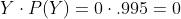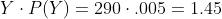Therefore, the expected payoff is$1.45. The expected profit of Raffle B is the expected payoff minus the cost: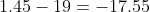Since -$14.50 > -$17.55, Raffle A has the better payoff.

### Example Question #73 : Probability

There are two raffles that both support causes that you care about. You have $20, and want to purchase a ticket for the raffle that gives you the best odds of winning. Raffle A supports a local charity, and has 100 tickets. Each ticket costs$17. One ticket will win a $110 prize, and the remaining tickets will win nothing. Raffle B supports a national charity, and has 200 tickets. Each ticket costs$19. One ticket will win a $410 prize, and the remaining tickets will win nothing. Which raffle is a better deal? Possible Answers: Raffle B, with payoff of -$15.90

Raffle A, with payoff of -$15.90 Raffle A, with payoff of -$16.95

Raffle B, with payoff of -$16.95 Correct answer: Raffle A, with payoff of -$15.90

Explanation:
To solve this problem, first calculate the expected profit of raffle A. The expected profit is the expected payoff minus the cost. Since it costs $17 to enter the raffle, the cost is$17. Next, let X be a random variable whose value is the payoff in the raffle. Since the only prize is $110, the values X can take are$110 and $0 (if no prize is won). Since 1 ticket has a payoff of$110, the probability of winning $110 is 1/100 = 0.01. In other words P($110)=.01. Since the 99 remaining tickets have a payoff of $0, the probability of winning$0 is P($0)=.99. To find E(X), the expected value of X, findfor each value k that X can take. Then sum these terms.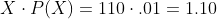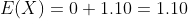Therefore, the expected payoff is$1.10. The expected profit of Raffle A is the expected payoff minus the cost: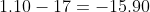Next, let's look at Raffle B. The cost of Raffle B is $19. Then, we want to find the expected payoff for Raffle B. This time, we'll use the random variable Y. Since the only prize is$410, the values X can take are $410 and$0 (if no prize is won). Since 1 ticket has a payoff of $410, the probability of winning$410 is 1/200 = 0.005. In other words P($410)=.005. Since the 199 remaining tickets have a payoff of$0, the probability of winning $0 is P($0)=.995.

To find E(Y), the expected value of Y, findfor each value k that Y can take. Then sum these terms.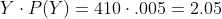Therefore, the expected payoff is $2.05. The expected profit of Raffle B is the expected payoff minus the cost: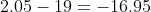Since -$15.90 > -\$16.95, Raffle A has the better payoff.

### Example Question #1 : Using Probability To Make Decisions

A spectator at a horse race is deciding which of three high-performing horses to bet on. Each horse’s ranks in past races can be expressed in terms of the following probability distributions: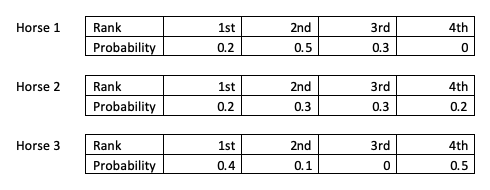The spectator wants to bet on the horse with the highest expected rank in its next race. Assuming that past performance is a good predictor of each horse’s performance in its respective next races, which horse should the spectator bet on?

Possible Answers:

Horse 2

Horse 3

Horse 1

Correct answer:

Horse 1

Explanation:

The expected value of each horse’s rank can be calculated as follows:

Expected value: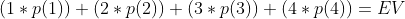Horse 1: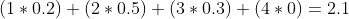Horse 2: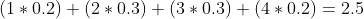Horse 3: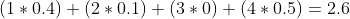The expected value predicts each horse’s rank in the upcoming race. Horse 1’s probability distribution yields the lowest expected value (i.e., the closest value to first place, represented by 1), so Horse 1 can be expected to rank most highly.

### Example Question #75 : Probability

A spectator at a horse race is deciding which of three high-performing horses to bet on. Each horse’s ranks in past races can be expressed in terms of the following probability distributions: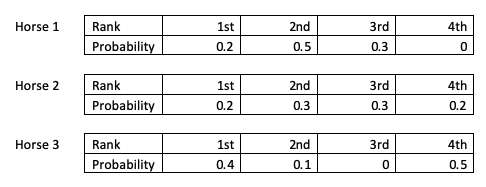After some calculation, the spectator identifies and bets on the horse with the highest expected rank in its next race. However, her chosen horse places last in its next race, the other two horses each place first in their next races, and the spectator loses her bet. Why did her strategy fail?

Possible Answers:

There's not enough information to answer this question

Chance

Probability was not calculated correctly

Correct answer:

Chance

Explanation:

Expected value identifies the most likely outcome, but other outcomes may still result, including unlikely outcomes. Although the horse the spectator bet on had the highest likelihood among the other horses of ranking well in its next race, the race was still ultimately subject to chance. Additionally, the three horses’ expected ranks differed only slightly--2.1, 2.5 and 2.6--meaning that the horse with the highest expected rank had only a slightly greater likelihood of performing more highly than the other two horses to begin with. As a result, chance was a relatively significant determinant of outcomes.

### Example Question #76 : Probability

Three students are playing a game with a fair six-sided die. If an even number is rolled, student A gets a point. If a number less than 4 is rolled, student B gets a point. If a prime number is rolled, student C gets a point. The die will be rolled fifteen times.

Is the game fair? In other words, do all three students have the same odds of getting a point?

Possible Answers:

No

Yes

Correct answer:

Yes

Explanation:

The sample space for the die roll is {1, 2, 3, 4, 5, 6}. Student A gets a point if 2, 4 or 6 is rolled. Student B gets a point if 1, 2 or 3 is rolled. Student C gets a point if 2, 3 or 5 is rolled. Therefore, all three students have a 3/6 = ½ = 0/5 = 50% chance of getting a point on each die roll. The number of times the die is rolled does not affect each student’s probability of getting a point; the probability is the same in each roll so long as neither the die nor the point rules change.

### Example Question #77 : Probability

An experimental drug is created to reduce the amount of time patients feel sick with the common cold. In clinical trials of people suffering from the common cold, different participants taking the drug experienced symptoms for varying lengths of time. The scientists running the trial rounded each participant’s duration of symptoms to the nearest day, and used this information to develop the following probability distribution: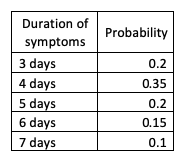There were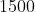participants. How many of them experienced symptoms for about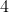days?

Possible Answers: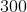participants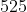participants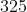participants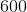participants

Correct answer:participants

Explanation:

If the probability distribution was constructed based on the real durations of participants’ symptoms, the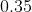probability corresponding to a-day duration of symptoms represents that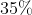of the total number of trial participants experienced symptoms for aboutdays. There wereparticipants, so the number of participants who experienced symptoms for aboutdays must have been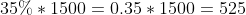participants.

### Example Question #78 : Probability

An experimental drug is created to reduce the amount of time patients feel sick with the common cold. In clinical trials of people suffering from the common cold, different participants taking the drug experienced symptoms for varying lengths of time. The scientists running the trial rounded each participant’s duration of symptoms to the nearest day, and used this information to develop the following probability distribution:If the scientists select one of the participants at random, what duration of symptoms can they expect the participant to have experienced?

Possible Answers: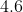days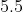days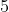days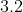days

Correct answer:days

Explanation:

The expected duration of a randomly selected patient’s symptoms can be calculated using the expected value yielded by this probability distribution. This expected value can be calculated as follows:

Expected value: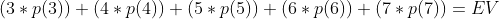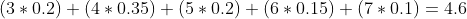Therefore, the most likely duration of symptoms a randomly selected participant would have isdays.

### Example Question #79 : Probability

A fair coin is flipped 9 times, yielding the following results: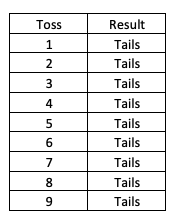Two students are deciding whether to flip this coin a tenth time, in order to decide which one of them will get to keep the five-dollar bill they found on a sidewalk. Would this be a fair method for making this decision?

Possible Answers:

No

Yes

Cannot be determined

Correct answer:

Yes

Explanation:

As long as the coin is truly fair, meaning that there is a 50% chance that it will land on heads and a 50% chance that it will land on tails, the coin will still be fair on the tenth flip. Past results do not affect the odds of a particular result on a subsequent identical event. Therefore, flipping this coin would be a fair way to decide which of the two students will get to keep the five-dollar bill, since each one of them would have a 50% probability of keeping the bill.

### Example Question #81 : Probability

Ann, Bob and Cathy are students working together on a group project for school. The project involves three tasks, each of which one of the three students will complete: creating a model, interviewing a local expert, and writing a report. No student has the time to complete more than one task, and all three of them have a strong preference for interviewing the local expert. They decide to find a fair way to randomly distribute the three tasks among themselves.

Which of the following would be a fair method of accomplishing this, allowing all three of them equal odds of completing their preferred task?

Possible Answers:

The three tasks are numbered, and the three resulting numbers are written on separate identical pieces of paper and put into a box. Each student takes turns drawing a piece of paper without looking and is assigned the task corresponding to their number.

Bob flips a coin. If it lands heads, he creates the model; if tails, he interviews a local expert. After he is assigned a task by this method, Cathy flips a coin. If it lands heads, she writes the report; if tails, she is assigned the other remaining task. Ann is then assigned whichever task is left.

The local expert chooses one of the three students.

Ann selects from the three tasks. Bob then chooses which he would prefer, and the remaining task is assigned to Cathy.

Correct answer:

The three tasks are numbered, and the three resulting numbers are written on separate identical pieces of paper and put into a box. Each student takes turns drawing a piece of paper without looking and is assigned the task corresponding to their number.

Explanation:

"Ann selects from the three tasks. Bob then chooses which he would prefer, and the remaining task is assigned to Cathy. " is incorrect because an intentional choice, particularly when biased by a strong preference for a single outcome, is unlikely to be random. Further, were Ann to choose to interview the expert, Bob and Cathy would never have a chance to be assigned that task, significantly advantaging one of the three students over the other two. "Bob flips a coin. If it lands heads, he creates the model; if tails, he interviews a local expert. After he is assigned a task by this method, Cathy flips a coin. If it lands heads, she writes the report; if tails, she is assigned the other remaining task. Ann is then assigned whichever task is left." is incorrect because Bob’s probability of interviewing the expert is 50% in this scenario, a higher probability than either Cathy or Ann would have. "The local expert chooses one of the three students." is incorrect because an intentional choice is unlikely to be random. "The three tasks are numbered, and the three resulting numbers are written on separate identical pieces of paper and put into a box. Each student takes turns drawing a piece of paper without looking and is assigned the task corresponding to their number. " is correct because any one of the three students may draw any one of the three slips, and cannot see which they are drawing, preventing their personal biases or preferences from influencing their selections.

← Previous 1

### All Algebra II Resources